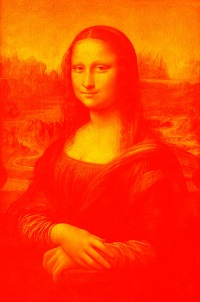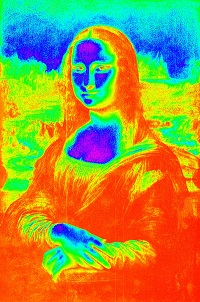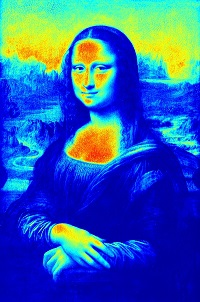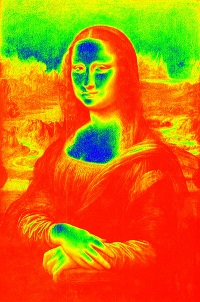# OpenCV - Color Maps

In OpenCV, you can apply different color maps to an image using the method applyColorMap() of the class Imgproc. Following is the syntax of this method −

```applyColorMap(Mat src, Mat dst, int colormap)
```

It accepts three parameters −

• src − An object of the class Mat representing the source (input) image.

• dst − An object of the class Mat representing the destination (output) image.

• colormap − A variable of integer type representing the type of the color map to be applied.

## Example

The following program demonstrates how to apply color map to an image.

```import org.opencv.core.Core;
import org.opencv.core.Mat;

import org.opencv.imgcodecs.Imgcodecs;
import org.opencv.imgproc.Imgproc;

public class ColorMapTest {
public static void main(String args[]) {

// Reading the Image from the file and storing it in to a Matrix object
String file ="E:/OpenCV/chap25/color_input.jpg";

// Creating an empty matrix to store the result
Mat dst = new Mat();

// Applying color map to an image
Imgproc.applyColorMap(src, dst, Imgproc.COLORMAP_HOT);

// Writing the image
Imgcodecs.imwrite("E:/OpenCV/chap25/colormap_hot.jpg", dst);
System.out.println("Image processed");
}
}
```

Assume that following is the input image color_input.jpg specified in the above program.## Output

On executing the above program, you will get the following output −

```Image Processed
```

If you open the specified path, you can observe the output image as follows −## More Operations

In addition to COLORMAP_HOT demonstrated in the previous example, OpenCV caters various other types of color maps. All these types are represented by predefined static fields (fixed values) of Imgproc class.

You can choose the type of the colormap you need, by passing its respective predefined value to the parameter named colormap of the applyColorMap() method.

```Imgproc.applyColorMap(src, dst, Imgproc.COLORMAP_HOT);
```

Following are the values representing various types of color maps and their respective outputs.

Operation and Description Output
COLORMAP_AUTUMNCOLORMAP_BONECOLORMAP_COOLCOLORMAP_HOTCOLORMAP_HSVCOLORMAP_JETCOLORMAP_OCEANCOLORMAP_PARULACOLORMAP_PINKCOLORMAP_RAINBOWCOLORMAP_SPRINGCOLORMAP_SUMMERCOLORMAP_WINTER